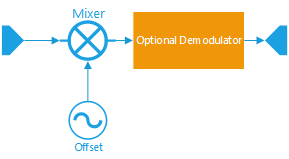## DDC

This object implements a Digital Down Converter (DDC) with the following features :

• Automatic configuration,
• Fractional convertion factors between input sample rate and output,
• Local numeric oscillator,
• Squelch,
• Support analog demodulator,
• AGC.`````` Object DDC {
constructor DDC( [String name] ); // create a new DDC object, the name is optional

bool setOutBandwidth( int Hz );   // configured the required output bandwidth
bool setCenter( float HZ ) ; // offset in HZ of the center of interest

bool write( IQData , [option:channel]);             // add a IQ block in the DDC

bool setSquelchLevel( number rmsdb, bool on_off  );
number getRMS() ;

bool setDemodulator( Demodulator object ); // add a demodulator
bool setAGC( bool on_or_off ) ;
}
``````

### .setOutBandwidth

Configure the DDC to output bandwidth.

``````slice.setOutBandwidth( output_band_Hz);
``````

### .setCenter

Configure the local oscillator frequency. I

`````` setCenter( Hz )
``````

### .write

Add IQ data to the DDC (input).
The DDC will reconfigure automatically itself to maintain the output bandwidth configued in setOutBandwidth( int Hz). The input block is processed and output is added to an internal FIFO. Internally, the DDC object uses the Overlap-Save method with a FFT. The output blocks should all have the same size, and the processing is only started when enough data has been added via the write() method. This means that each call to .write() does not produce a output block.

``````var iq_out =  write( IQBlock data_in, [option:channel] );
``````

Extract a resulting block from the DDC.

``````slice.read( IQdata );
``````

### .setSquelchLevel

``````.setSquelchLevel(rmsdb,on);
``````

### .getRMS

Returns the RMS level, actually 10*log10( rms power )

### .setDemodulator

Configure the DDC to call the given demodulator object and then return in read() the demodulator output instead of the IQ complex output.

Note : for compatibility reason, the output of the DDC will remain complex (IQData objects), even if the demodulator should output real samples. The IQData returned from the DDC using a demodulator as its real and imaginary part equal. You may retrieve the demodulated output using getReal() for example.

Compatible demodulators :

``````  var FMdemod = new NBFM('fm');
FMdemod.configure(  {'modulation_index': 0.2} );

var ddc = new DDC();
ddc.setCenter( 100e3 );
ddc.setOutBandwidth( 12e3 );
ddc.setDemodulator( FMdemod ); // tells the DDC to call the FMDemod object before output

for( ; ; ) {
...
if( IQBlock.readFromQueue( fifo_from_rx ) ) {
ddc.write( IQBlock);
var fm_audio = ddc.read(); // output is IQData !!
...
}
}
``````

### Example

Full example using a FIFO RX as input, local file as output :

``````/*
[RX] ==> [queue ] ==> extract a suddand of 48 kHZ BW @ +176 kHz from center ==> [queue] ==> [file]
*/
// create working queues and objects
var fifo_from_rx = Queues.create( 'input');
var fifo_to_file = Queues.create( 'outut');
var IQBlock = new IQData('iq');
var samples = 0 ;

// open RX
var rx = Soapy.makeDevice( {'query' : 'driver=rtlsdr' });
if( typeof rx != 'object' ) {
exit();
}

if( !rx.isValid()) {
exit();
}

if( rx.isAvailable() ) {
// set sample rate
if( rx.setRxSampleRate( 1e6 )) {
print('Sample rate changed');
}
} else {
print('device is already used, we do not change Sampling Rate');
}

rx.setRxCenterFreq( 466 );

// create output file
print('create out queue');
fifo_to_file.writeToFile('/tmp/rx.cf32');

// engage streaming
if( !fifo_from_rx.ReadFromRx( rx ) ) {
print('Cannot stream from rx');
exit();
}

var slice = new DDC('one');
slice.setOutBandwidth(48e3); // 48 kHz output
slice.setCenter( 176e3 ) ; // receive 48kHz centered at +176 kHz from center

print('starting rx process');
while( fifo_from_rx.isFromRx() ) { // if we have something in the input
if( IQBlock.readFromQueue( fifo_from_rx ) ) {     // load samples from input queue into IQBlock object
slice.write( IQBlock );               // write the samples in the DDC object